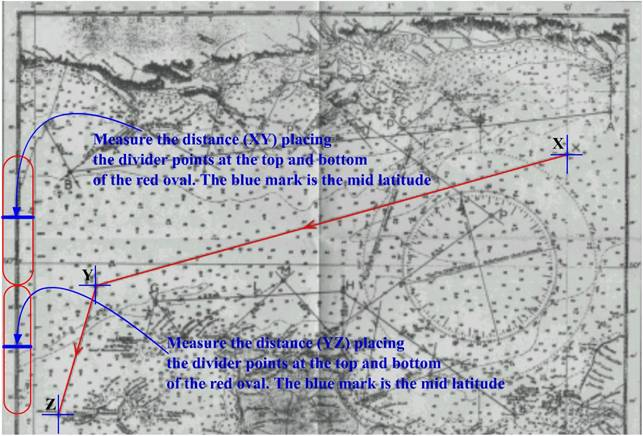Back to Nautical Definition Charts Rotation of the Earth Distances Position Lines Sailings Chart Work Exercises Information from Charts Tides Sextant

Distances

Approximate polar and equatorial circumferences of the earth

A number of reference ellipsoids are in use to describe the shape of the earth, for example the World Geodetic System (WGS) ellipsoid of 1984.

Earth data (WGS 84 ellipsoid):

Equatorial radius (Re) 6378137.0 m     Circumference as: 40080212.908m

Polar radius (Rp) 6356752.3142 m      Circumference as: 39945831.542m

Flattening ((Re – Rp) / Re) 1/298.25722

The values for polar radius and equatorial radius of the earth, the great circle distance of 1’ is 1.849 km at the poles and 1.855 km at the equator. This small difference does not produce a significant error when plotting lines of position. It is therefore sufficient to use the adopted mean value (1 nautical mile = 1.852 km).

However, when calculating the great circle distance of two locations thousands of nautical miles apart, the error caused by the flatness of the earth can increase to several nautical miles.

Measuring the distance between two positions on a Mercator chart

Measure distances using the chart’s latitude scale. Assuming that one-minute of latitude equals one nautical mile introduces no significant error. Since the Mercator’s latitude scale expands as latitude increases, measure distances on the latitude scale closest to the area of interest. On large scale charts, such as harbour charts, use the distance scale provided. To measure long distances on small-scale charts, break the distance into a number of segments and measure each segment at its mid-latitude.